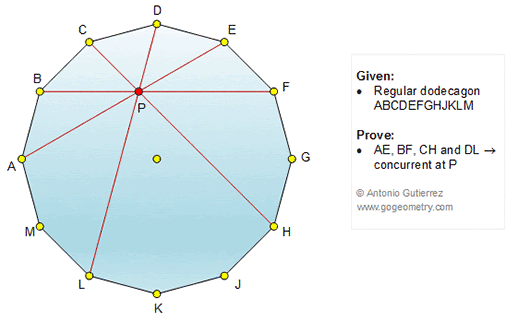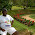Friday, June 29, 2018

Geometry Problem 1358: Regular Dodecagon, Diagonal, Concurrency, Concurrent Lines

Geometry Problem. Post your solution in the comment box below.
Level: Mathematics Education, High School, Honors Geometry, College.

Details: Click on the figure below.1.P the point BF meet AE, join P to L, H, C, D
Tr APB = tr PEF (ASA) => tr APL = tr PFH (SAS)
Draw PH, PH1 altitudes for PLH and tr PCD => H, P, H1 collinear
=> L, P, D collinear

2.https://photos.app.goo.gl/fN9T3nP2Xm81pZ8YA

Draw BF, CH , PA , PL and diameter BH and AG
Typical Arc(AB) = 360/12 =30 degrees
1. Triangle OFH is equilateral and triangle BHF is a 30-60-90 triangle
PFH is 45-45-90 triangle and PF=FH=OF=OA=AL
Quadrilateral AOFP is a rhombus ( OA=PF, OA//PF and OA= OF=PF)
So ∠ (PAG)= ∠ (PFO)= 30 degrees = ∠ (GAE) => A, P, E are collinear
2. Since A,P,E are collinear => ∠ (EAL)= 90 and ALP is a 45-45-90 triangle
And ∠ (ALP)=45 degrees= ∠ (ALD) => L,P, D are collinear
So BF,CH, AE and LD are concurrent at P

3.CH, DL, BH are angle bisectors of triangle BDH, while DL, CH, AE are altitudes of triangle ADH.

1.Excellent solution Stan